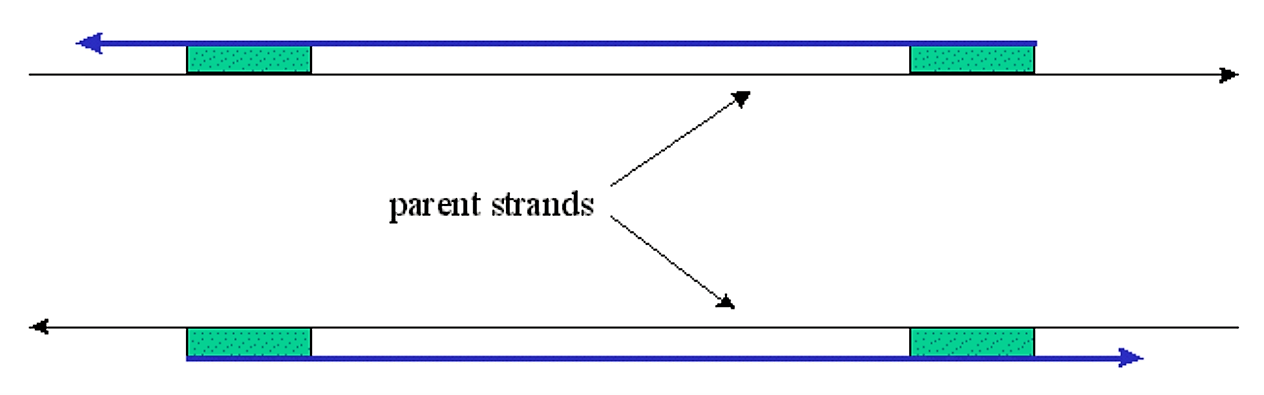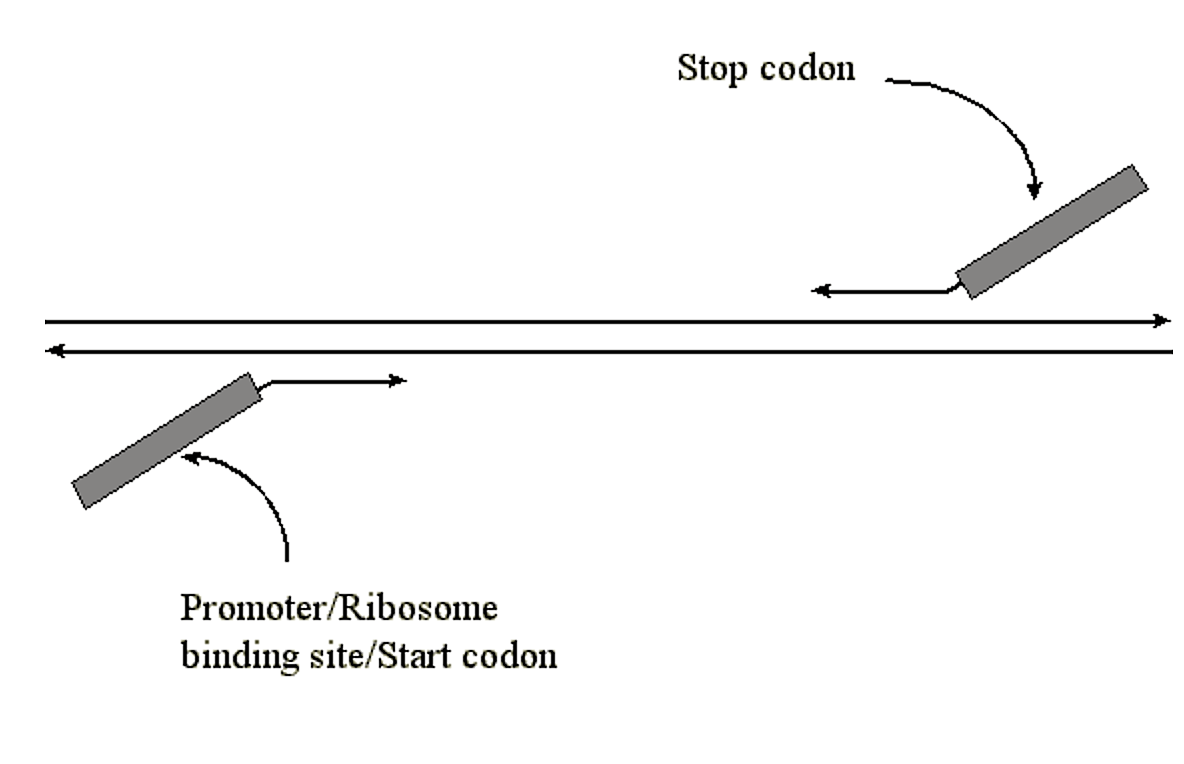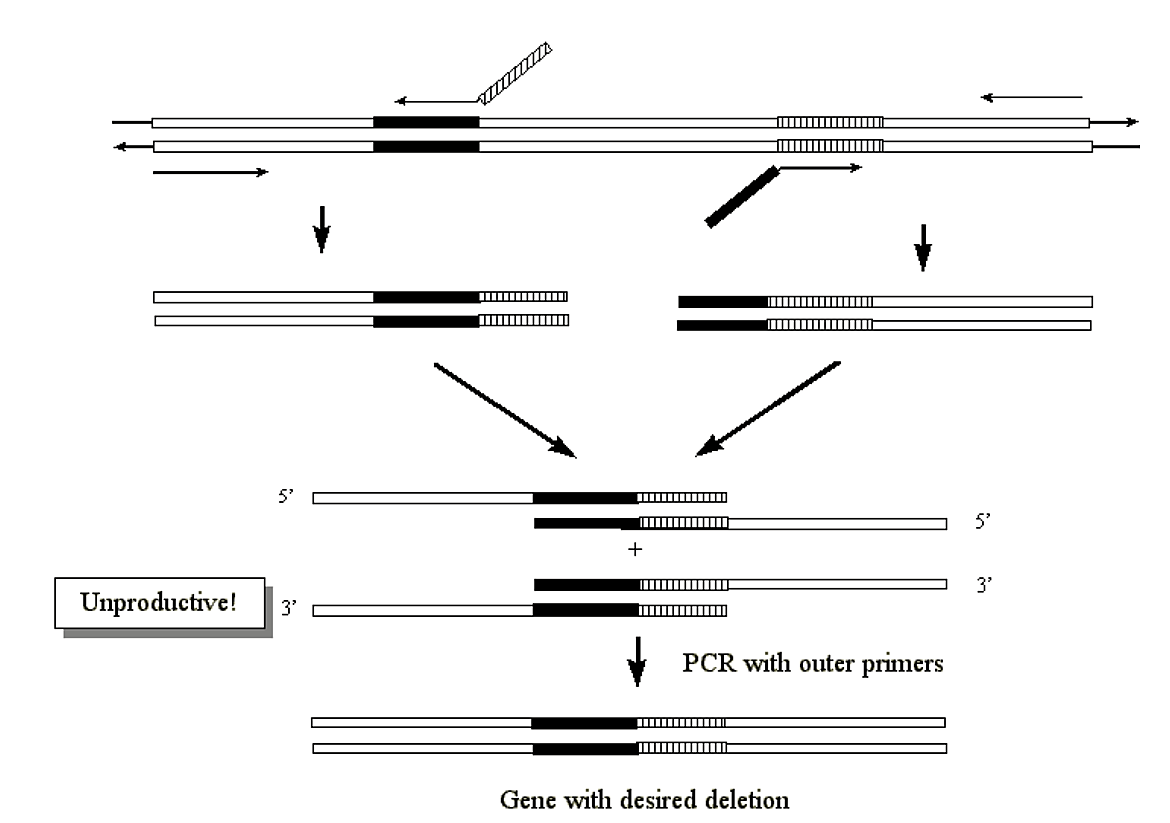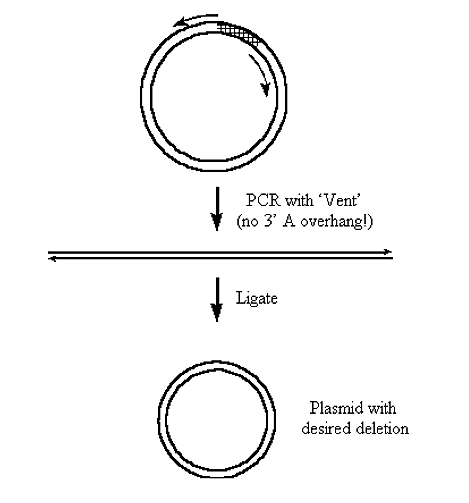# 3.3: Polymerase Chain Reaction (PCR) and Cloning of PCR Products

$$\newcommand{\vecs}{\overset { \rightharpoonup} {\mathbf{#1}} }$$ $$\newcommand{\vecd}{\overset{-\!-\!\rightharpoonup}{\vphantom{a}\smash {#1}}}$$$$\newcommand{\id}{\mathrm{id}}$$ $$\newcommand{\Span}{\mathrm{span}}$$ $$\newcommand{\kernel}{\mathrm{null}\,}$$ $$\newcommand{\range}{\mathrm{range}\,}$$ $$\newcommand{\RealPart}{\mathrm{Re}}$$ $$\newcommand{\ImaginaryPart}{\mathrm{Im}}$$ $$\newcommand{\Argument}{\mathrm{Arg}}$$ $$\newcommand{\norm}{\| #1 \|}$$ $$\newcommand{\inner}{\langle #1, #2 \rangle}$$ $$\newcommand{\Span}{\mathrm{span}}$$ $$\newcommand{\id}{\mathrm{id}}$$ $$\newcommand{\Span}{\mathrm{span}}$$ $$\newcommand{\kernel}{\mathrm{null}\,}$$ $$\newcommand{\range}{\mathrm{range}\,}$$ $$\newcommand{\RealPart}{\mathrm{Re}}$$ $$\newcommand{\ImaginaryPart}{\mathrm{Im}}$$ $$\newcommand{\Argument}{\mathrm{Arg}}$$ $$\newcommand{\norm}{\| #1 \|}$$ $$\newcommand{\inner}{\langle #1, #2 \rangle}$$ $$\newcommand{\Span}{\mathrm{span}}$$$$\newcommand{\AA}{\unicode[.8,0]{x212B}}$$

PCR is an in vitro technique for the amplification of a region of DNA which lies between two regions of known sequence. PCR amplification is achieved by using oligonucleotide primers. These are typically short, single stranded oligonucleotides which are complementary to the outer regions of known sequence.Figure 3.3.1: Complementary oligonucleotide primers

The oligonucleotides serve as primers for DNA polymerase and the denatured strands of the large DNA fragment serves as the template. This results in the synthesis of new DNA strands which are complementary to the parent template strands. These new strands have defined 5' ends (the 5' ends of the oligonucleotide primers), whereas the 3' ends are potentially ambiguous in length.Figure 3.3.2: Synthesis of new DNA strands

The oligonucleotide directed synthesis of daughter DNA strands can be repeated if the new duplex is denatured (by heating) and additional primers are allowed to anneal (by cooling to an appropriate temperature).

The steps of:

1. Template denaturation
2. Primer annealing
3. Primer extension

comprise a single "cycle" in the PCR amplification methodology.

After each cycle the newly synthesized DNA strands can serve as templates in the next cycle.Figure 3.3.3: Product of elongation reaction as template

Note that half of the newly synthesized strands from the second round of replication have 5' and 3' termini which are defined by the annealing location of the primer oligonucleotides.

Summary of products at the end of each PCR cycle:Figure 3.3.4: PCR products

The total of each of the different types of oligonucleotide fragments for each cycle can be summarized as follows:Figure 3.3.5: Types of fragments for each cycle

• The amplification of the fragments follows the following pattern:
• There is always 1x copy of each of the original templates - the PCR as outlined never reproduces the full length template
• There will be 'n'x copies of each fragment with an indeterminate length (where 'n' is the number of cycles). The fragments of indeterminant length have one end defined by a PCR primer and the other end is of indeterminant length
• There will be (2n - (n + 1)) x copies of each fragment of defined length (i.e. each end defined by the two PCR primers).
• For example, after 6 cycles we will have
• 1 x copy of each original template
• 6 x copies of each fragment of indeterminant length
• (64 - (6 + 1)) = 57 x copies of each fragment of defined length
• In the above case, the desired PCR product will be a duplex of the defined length fragment. The question is: how many will be produced?
• The original templates will not necessarily anneal with one another
• Likewise, the templates of indeterminant length will not necessarily anneal with one another
• Furthermore, as the number of cycles proceeds, the defined length fragments far outnumber the others
• Therefore, the original template, and fragments of indeterminant length are most likely going to hybridize with defined length fragments
• Our expected amplification would therefore be (using the 6 cycle PCR example)

57 - (6 + 1) = 50 x copies of each defined length fragments

(i.e. 50 x duplexes of defined length)

• The expected amplification of the desired defined length product with respect to the original template concentration ($$x$$) can thus be represented by the formula:

$[(2^n - (n + 1)) - (n + 1)] x$

or

$(2^n - 2(n + 1))$

(this is often abbreviated to a simple rule of thumb for the amplification: (2n - 2n) x)

The interpretation of this formula is that

• For a given number of cycles 'n' we make '2n x' total possible duplexes
• For a given number of cycles there will be '2(n+1) (or 2n in our approximation) x' duplexes which are formed from either the original template, or a fragment of indeterminate length, along with a fragment of defined length (and represent an undesired product)
• Thus, the total concentration of desired product (duplexes with a length defined by the PCR primers) will be
(2n - 2(n+1)) x (where x is the concentration of the original duplex)
• The theoretical amplification value is never achieved in practice. Several factors prevent this from occurring, including:
1. Competition of complementary daughter strands with primers for reannealing (i.e. two daughter strands reannealing results in no amplification).
2. Loss of enzyme activity due to thermal denaturation, especially in the later cycles
3. Even without thermal denaturation, the amount of enzyme becomes limiting due to molar target excess in later cycles (i.e. after 25 - 30 cycles too many primers need extending)
4. Possible second site primer annealing and non-productive priming

Note

PCR was invented in 1985 by Kary Mullis, working for Cetus corporation somewhere near Berkeley, California. The original method of PCR used the Klenow fragment of E. coli DNA polymerase I. This enzyme, however, denatures at temperatures lower than that required to denature most template duplexes. Thus, after each cycle, fresh enzyme had to be added to the reaction. This was quite tedious. In addition to this problem with the enzyme, the samples had to be moved from one temperature bath to another to allow the individual steps of denaturation, annealing and polymerization, which all required different temperatures. This was pretty tedious too.

Thus, two main advances allowed the process to be automated, these advances were:

1. The use of thermostable DNA polymerases, which resisted denaturation (inactivation) at high temperatures. Thus, an initial aliquot of polymerase could last throughout the numerous cycles of the protocol. The first thermostable DNA polymerase to be used was isolated from the bacterium Thermus aquaticus. It was isolated from a hot spring in Yellowstone National Park where it lived happily (i.e. it replicated its DNA) at temperatures in excess of 85 °C
2. The development of temperature baths which could shift their temperatures up and down rapidly and in an automated programmed manner. These are known as thermal cyclers or PCR machines.

These two developments let to the automation of PCR. The PCR process is covered by patents owned by Hoffmann-La Roche Inc. (a faceless conglomerate you can trust).

### Thermal cycling parameters

• The thermal cycling parameters are critical to a successful PCR experiment. The important steps in each cycles of PCR include:
1. denaturation of template
2. annealing of primers
3. extension of the primers

A representative temperature profile for each cycle might look like the following:Figure 3.3.6: Temperature for PCR cycle

#### Template denaturation

• The initial denaturation of template is accomplished at 95-100 °C.
• Supercoiled plasmids are tougher to melt and may require boiling for several minutes, or may be initially denatured by using base (NaOH, followed by pH neutralization).
• Denaturation during the PCR experiment (i.e. second cycle onward) is usually accomplished at temperatures of 92-95 °C (usually empirically determined).

#### Primer annealing temperature

• Primer annealing temperature is an important parameter in the success of the PCR experiment.
• The annealing temperature is characteristic for each oligonucleotide:
• it is a function of the length and base composition of the primer as well as the ionic strength of the reaction buffer.
• Estimates of the annealing temperature can be calculated in several different ways.
• These calculated annealing temperatures are a starting point for the PCR experiment, but ideal annealing temperatures are determined empirically.

#### Primer extension

• Primer extension is usually performed at 72 °C, or the optimum temperature of the DNA polymerase.
• The length of time of the primer extension steps can be increased if the region of DNA to be amplified is long, however, for the majority of PCR experiments an extension time of 2 minutes is sufficient to get complete extension.

#### Number of cycles

• The number of cycles is usually between 25 and 35.
• More cycles mean a greater yield of product.
• However, with increasing number of cycles the greater the probability of generating various artifacts (e.g. mispriming products).
• It is unusual to find procedures which have more than 40 cycles.

### Choice of Polymerases for PCR

• One of the important advances which allowed development of PCR was the availability of thermostable polymerases.
• This allowed initially added enzyme to survive temperature cycles approaching 100 °C.

Thermostable DNA polymerases and their sources

DNA Polymerase

Natural or recombinant

Source

Taq

Natural

Thermus aquaticus

Amplitaq®

Recombinant

T. aquaticus

Amplitaq (Stoffel fragment)®

Recombinant

T. aquaticus

Hot Tub™

Natural

Thermus flavis

Pyrostase™

Natural

T. flavis

Vent™

Recombinant

Thermococcus litoralis

Deep Vent™

Recombinant

Pyrococcus GB-D

Tth

Recombinant

Thermus thermophilus

Pfu

Natural

Pyrococcus furiosus

ULTma™

Recombinant

Thermotoga maritima

• Properties of DNA polymerases used in PCR

Taq/Amplitaq®

Stoffel fragment

Vent™

Deep Vent™

Pfu

Tth

ULTma™

95 °C half-life

40 min

80 min

400 min

1380 min

>120 min

20 min

>50 min

5'3' exo

+

+

3'5' exo

+

+

+

+

Extension rate (nt/sec)

75

>50

>80

?

60

>33

?

RT activity

Weak

Weak

?

?

?

Yes

?

Resulting ends

3' A

3' A

>95% blunt

>95% blunt

blunt

3' A

blunt

Strand displacement

+

+

M.W. (kDa)

94

61

?

?

92

94

70

### Buffers and MgCl2 in PCR reactions

A typical reaction buffer for PCR would something like:

• 10 mM Tris, pH 8.3
• 50 mM KCl
• 1.5 mM MgCl2
• 0.01% gelatin
• The MgCl2 concentration in the final reaction mixture is usually between 0.5 to 5.0 mM, and the optimum concentration is determined empirically (typically between 1.0 - 1.5 mM). Mg2+ ions:
• form a soluble complex with dNTP's which is essential for dNTP incorporation
• stimulate polymerase activity
• increase the Tm (melting temperature) of primer/template interaction (i.e. it serves to stabilize the duplex interaction

Generally,

• low Mg2+ leads to low yields (or no yield) and
• high Mg2+ leads to accumulation of nonspecific products (mispriming).

### Primers

Primer design

• Generally, primers used are 20 - 30 mer in length. This provides for practical annealing temperatures (of the high temperature regimen where the thermostable polymerase is most active).
• Primers should avoid stretches of polybase sequences (e.g. poly dG) or repeating motifs - these can hybridize with inappropriate register on the template.
• Inverted repeat sequences should be avoided so as to prevent formation of secondary structure in the primer, which would prevent hybridization to template
• Sequences complementary to other primers used in the PCR should be avoid so as to prevent hybridization between primers (particularly important for the 3' end of the primer)
• If possible the 3' end of the primer should be rich in G, C bases to enhance annealing of the end which will be extended
• The distance between primers should be less than 10 Kb in length. Typically, substantial reduction in yield is observed when the primers extend from each other beyond ~3 Kb.

#### Melting temperature (Tm) of primers

• The Tm of primer hybridization can be calculated using various formulas. The most commonly used formula is:

$Tm = [(number of A+T residues) \times 2 °C] + [(number of G+C residues) x 4 °C]$

• This formula was determined originally from oligonucleotide hybridization assays, which were performed in 1 M NaCl, and appears to be accurate in lower salt conditions only for primers less than about 20 nucleotides in length.
• The common wisdom is that the Tm is more like 3-5 °C lower than the value calculated from this formula.

$Tms = 81.5 + 16.6(\log_{10}[J+]) + 0.41(\%G+C) - (600/l)$

• Where [J+] = the molar concentration of monovalent cations (e.g. Na+ from NaCl), and l = the length of oligonucleotide. (%G+C) is the actual percentage value and not a fractional representation (i.e. the value to insert for a primer which had 90 % G+C content would be "90" and not "0.90").
• This formula is reportedly useful for primers of 14 to 70 bases in length.

$Tmp = 22 + 1.46([2 \times (G+C)] + (A+T))$

• This formula is reportedly useful for primers of 20-35 bases in length.
• The calculated annealing temperature is only a reference temperature from which to initiate experiments.
• The actual annealing temperature may be 3-12 °C higher than the calculated Tm.
• The actual annealing temperature condition should be determined empirically.
• The highest annealing temperature which gives the best PCR product should be used.
• Examples of Tm , Tms and Tmp calculations (0.05 M K+)

G

A

T

C

Tm

Tms

Tmp

15 mer

3

5

2

5

46

42

56

20 mer

6

5

4

5

62

52

67

30 mer

8

6

8

8

92

62

89

#### Calculating primer concentrations

• The molar concentration of a primer can be calculated based on the absorbance of the primer at 260 nm (A260) and the molar extinction coefficient for the primer at this wavelength.
• The molar extinction coefficient for the primer can be calculated by knowing the sequence of the primer and then summing the molar extinction values for the individual bases which comprise the primer.
• The individual bases have the following molar extinction coefficients at 260 nm:
• A 1.0 molar solution of dT has a value of 8,400 absorbance units at 260 nm.
• A 1.0 molar solution of dA has a value of 15,200 absorbance units at 260 nm.
• A 1.0 molar solution of dG has a value of 12,010 absorbance units at 260 nm.
• A 1.0 molar solution of dC has a value of 7050 absorbance units at 260 nm.
• For example, the primer 5' TAGC 3' would have a molar extinction coefficient of 42,660 at 260 nm. Likewise, a 10 micromolar solution of this primer would give an absorbence of 0.427 at 260 nm.

## Cloning PCR Products

### Introduction of restriction sites

• It is possible to introduce restriction site sequences into PCR products by having these sequences incorporated into the 5' end of the PCR primer(s).Figure 3.3.7: Restriction site sequence introduction

• The short restriction site sequence on the 5' end of the PCR primer will not hybridize, but as long as the 3' hybridizing region is long enough (i.e. its Tm is high enough; ~20 mer) the primer will specifically bind to the appropriate site.
• The PCR product will thus have an additional DNA squence at the 5' end which will contain the endonuclease restriction site.
• A similar or different restriction site sequence can be added via the other PCR primer.
• If the other primer has a different restriction sequence then the PCR fragment can be inserted in a directional dependent manner in a host plasmid.

The potential problems with this method include:

• There is no easy way to prevent internal sites containing similar restriction sequences from being cut when the end of the PCR product are cut
• Restriction sequences are inverse repeat sequences, thus the potential exists for primer dimer association and resultant non-productive annealing

### Generation of half sites

• This method is similar to the method of introducing restriction sites, described above.
• The primary difference is that instead of the primer containing the entire restriction site sequence (say the six nucleotides of a six cutter) it will contain only the last three (and the other PCR primer will contain the complementary sequence for the first three).Figure 3.3.8: Half sites

The advantages of this method are:

• Typically internal restriction sites cleave with much greater efficiency (i.e. some sites if located at the ends of linear DNA never cut well at all)
• There is no need to gel purify linker fragments after digestion
• The DNA can be methylated (the half sites will not be). After concatenation the linkers will be cut but internal restriction sites will not
• A disadvantage is that the same restriction site is incorporated into both ends so the PCR fragment cannot be ligated into a host vector in an orientation dependent manner.
• Also, in this method 3' A overhang cannot be tolerated.

Blunt end ligation

• As stated earlier some thermostable DNA polymerases add a single dA residue onto the 3' end of the PCR product.
• There are three choices to be made when attempting to subclone without the use of added restriction sites within the primers:
• Use a DNA polymerase which leaves the 3' strand blunt (e.g. Vent) and do a blunt end ligation (i.e. host vector was opened up with blunt cutting restriction endonuclease)
• "Fix" the 3' A overhang by chewing back with Pol I, dNTP's.
• Use the 3' A overhang to anneal and ligate to a "T" vector - a vector which has a single dT overhang on its 3' ends.
• "T" vectors can be made by opening up a vector, via a blunt cutting restriction endonuclease and ligating in a specific linker.
• The linker contains a restriction sequence for a restriction endonuclease which recognizes an interrupted palindrome and cuts in the internal region with a single 3' overhang.
• The linker will contain two copies of the restriction recognition sequence, the first with a sequence in the interrupted palindrome which leaves a 3' T overhang and the second with a sequence in the interrupted palindrome which leaves another 3' T overhang
• This may sound confusing, so here's an example of how to make a T vector:

Ahd I restriction endonuclease:

           |
G A C N N N N N G T C
C T G N N N N N C A G
|


Ahd I will cut this sequence to produce:

G A C N N N                 N N G T C
C T G N N                 N N N C A G


We could design an oligonucleotide with two Ahd I restriction sequences, with slightly different sequences in the interrupted region of the palindrome, to give:

           |                     |
G A C N N T N N G T C G A C N N A N N G T C
C T G N N A N N C A G C T G N N T N N C A G
|                     |


If this this were inserted into a vector, and the vector then was cut with Ahd I, it would have the following sequence at the ends of the linearized vector:

-G A C N N T                       N N G T C-
-C T G N N                       T N N C A G-


In other words, a 3' 'T' overhang at both ends of the vector. A PCR product, with 3' A overhangs could thus be inserted into such a 'T' vector

### Adding promoters, ribosome binding sites, start codons, and stop codons

• The ability to add unique sequences to the 5' ends of PCR primers allows for short control elements to be directly incorporated.
• These can include a start codon or stop codon (3 bases), a promoter (~30 nucleotide region) or a ribosome binding site (~8 bases).Figure 3.3.9: Inserting promoter/ribosome/binding site/start codon/stop codon

### PCR Mutagenesis

#### Gene fusion

• This method is useful for joining overlapping regions of a large gene, or for the construction of chimeric genes.Figure 3.3.10: Gene Fusion

#### Creation of deletions within a gene

• A very similar methodology can be used within a single gene for the production of a mutant gene containing a specific deletion:Figure 3.3.11: Deletions

• If the gene is contained within circular DNA (i.e. a plasmid) deletions can be constructed in a single PCR reaction with a single set of primers (this type of methodology is also known as "inverse" PCR).Figure 3.3.12: Inverse PCR

#### Generation of point mutation(s) - i.e. base substitution mutations

• The generation of base substitutions can proceed along a similar route as with the deletion mutations.
• However, in this case the primers are mutagenic - there will be a mismatch, or mismatches, between the primer and target sequences.
• The mutagenic oligo will have a lower than expected Tm due to this mismatch(es).Figure 3.3.13: Point mutation

#### Introduction of base substitutions via asymmetric PCR:Figure 3.3.14: Substitution with asymmetric PCR

#### Insertion mutagenesis

• Short insertions (~1-6 basepairs) can be incorporated directly into a PCR primer, either internally, or at the 5' end.
• If the template DNA is linear and the desired site of insertion is not at the end of the template, then the entire gene (plus insertion) can be produced using asymmetric PCR or overlapping PCR (i.e. shown above).Figure 3.3.15: Insertion mutagenesis

• Large insertions can be accomplished by using a template (the desired insertion) for PCR with the primers having 5' sequences which are complementary to the region of insertion in the desired gene:Figure 3.3.16: Large insertion

#### "Random" mutagenesis with PCR

• The PCR protocol can be modified so as to introduce mutations at random positions in the target DNA.
• The principle behind the mutagenesis is misincorporation of bases at "random" positions.
• Misincorporation by Taq polymerase, for example, can be achieved by adding Mn2+ to the reaction buffer, and decreasing the concentration of one of the four dNTP's.
• At the sites in the template where the reduced base should be incorporated, there will be an increased probability of misincorporation.
• Thus, the choice of base with diminished concentration determines the sites in the template which will potentially be mutated.
• The misincorporated base is more or less random.
• The ideal Mn2+ concentration to add varies between 0.1 to 0.5 mM and is determined empirically. The relative concentrations of bases is 1 mM for each base, except the reduced base, which is typically present at a 1:5 or 1:10 ratio (i.e. 0.2 to 0.1 mM).

This page titled 3.3: Polymerase Chain Reaction (PCR) and Cloning of PCR Products is shared under a not declared license and was authored, remixed, and/or curated by Michael Blaber.